# ISEE Upper Level Quantitative : How to multiply variables

## Example Questions

← Previous 1 3

### Example Question #1 : How To Multiply Variables

Multiply: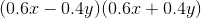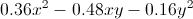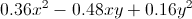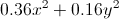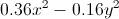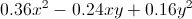Explanation:

This can be solved using the pattern for the sum times difference of two terms: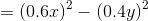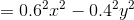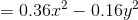### Example Question #1 : Operations

Multiply: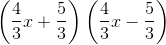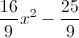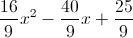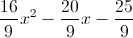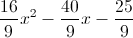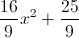Explanation:

This can be solved using the pattern for the sum times difference of two terms: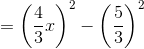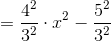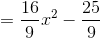### Example Question #1 : How To Multiply Variables

Simplify: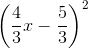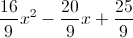Explanation:

This can be solved using the pattern for the square of a binomial: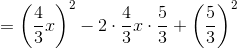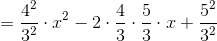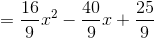### Example Question #2 : Operations

N is a positive integer;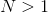. Which is greater?

(a)(b) 0

(a) and (b) are equal

It is impossible to tell from the information given

(a) is greater

(b) is greater

(a) is greater

Explanation: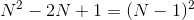. Since,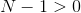, and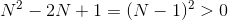. This makes (a) greater.

### Example Question #3 : Operations

N is a positive integer;. Which is greater?

(a)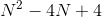(b)(a) and (b) are equal.

(b) is greater.

(a) is greater.

It is impossible to tell from the information given.

It is impossible to tell from the information given.

Explanation:

If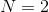, then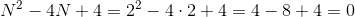.

If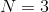, then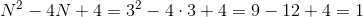.

Therefore, at least two possibilities can be demonstrated.

### Example Question #4 : Operations

Which is the greater quantity?

(a)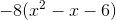(b)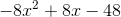(b) is greater.

It is impossible to tell from the information given.

(a) and (b) are equal.

(a) is greater.

(a) is greater.

Explanation: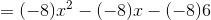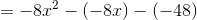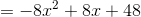Since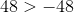,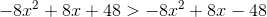.

### Example Question #7 : How To Multiply Variables

Assumeis nonzero. Which expression is not equivalent to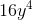?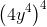All four expressions given in the other choices are equivalent to.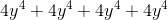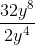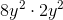Explanation:

Each expression can be simplified using the properties of exponents, among others: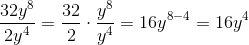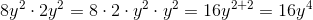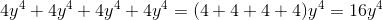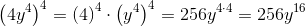The correct choice is, as it is the only choice not equivalent to.

### Example Question #5 : Operations

Letbe negative. Which of the following is the greater quantity?

(A)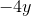(B)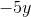It is impossible to determine which is greater from the information given

(A) is greater

(A) and (B) are equal

(B) is greater

(B) is greater

Explanation: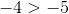,

So by the multiplication property of inequality, when each is multiplied by the negative number,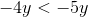and (B) is greater.

### Example Question #851 : Isee Upper Level (Grades 9 12) Quantitative Reasoningis a positive number. Which is greater?

(A) One ninth of 117% of(B) One eleventh of 143% of(B) is greater

It is impossible to determine which is greater from the information given

(A) is greater

(A) and (B) are equal

(A) and (B) are equal

Explanation:

117% ofis equal to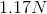.

One-ninth of this is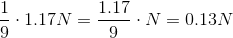143% ofis equal to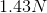One-eleventh of this is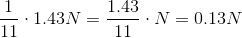Regardless of the value of, the quanitites are equal.

### Example Question #6 : Operations

Which of the following expressions is equivalent to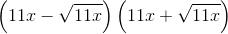?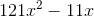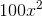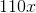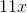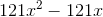Explanation:

Use the difference of squares pattern as follows: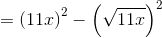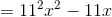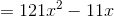← Previous 1 3